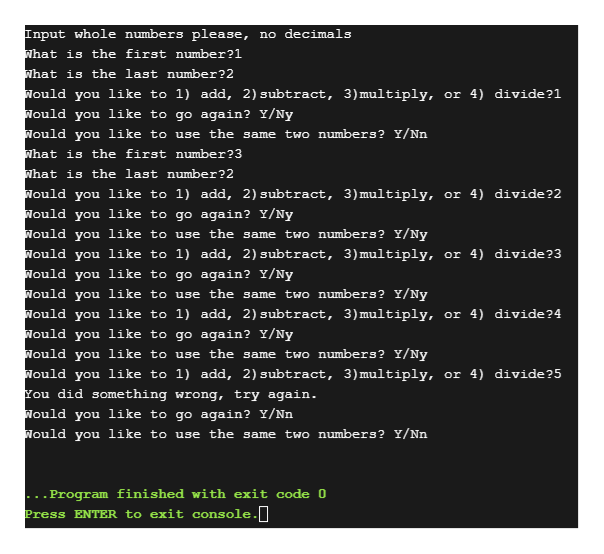Question
47 views

Calculator Challange

Python Coding

Create a simple calculator that takes in TWO numbers from the user and then asks the user to select if they want to ADD, SUBTRACT, MULTIPLY or DIVIDE to get the answer.

I have coded...

print("input whole numbers please, no decimals")
again=""
while again!="N":
first=int(input("What is the first number?"))
last=int(input("What is the last number?"))
#let user pick the operation, assign numbers to the operations
operation=int(input("Would you like to 1) add, 2)subtract, 3)multiply, or 4) divide?"))
if operation==1:
elif operation==2:
elif operation ==3:
elif operation == 4:
else:
print("You did something wrong, try again.")
again=str(input("Would you like to go again? Y/N")).upper()
same=str(input("Would you like to use the same two numbers? Y/N")).upper()
while same=="Y":
operation = int(input("Would you like to 1) add, 2)subtract, 3)multiply, or 4) divide?"))
if operation == 1:
elif operation == 2:
elif operation == 3:
elif operation == 4:
else:
print("You did something wrong, try again.")
again = str(input("Would you like to go again? Y/N")).upper()
same = str(input("Would you like to use the same two numbers? Y/N")).upper()

Finish the coding to...

-the numbers inputted and the answers are written into a text file

-output to the text file the full sentence of what operations you are doing.  For example output to the text file :

2 x 3 = 6
check_circle

Step 1

A Python program for the given criteria is as follows,

File name : “main.py”

print("Input whole numbers please, no decimals")

again=""

#input("Do you want to continue Y/N")

while again!="N":

first=int(input("What is the first number?"))

last=int(input("What is the last number?"))

#let user pick the operation, assign numbers to the operations

operation=int(input("Would you like to 1) add, 2)subtract, 3)multiply, or 4) divide?"))

if operation==1:

elif operation==2:

elif operation ==3:

elif operation == 4:

else:

print("You did something wrong, try again.")

again=str(input("Would you like to go again? Y/N")).upper()

same=str(input("Would you like to use the same two numbers? Y/N")).upper()

while same=="Y":

operation = int(input("Would you like to 1) add, 2)subtract, 3)multiply, or 4) divide?"))

if operation == 1:

elif operation == 2:

elif operation == 3:

elif operation == 4:

else:

print("You did something wrong, try again.")

again = str(input("Would you like to go again? Y/N")).upper()

same = str(input("Would you like to use the same two numbers? Y/N")).upper()

Screenshot of code #1:

Step 2

Screenshot of code #2:

Step 3

Screenshot of console outp...help_outlineImage TranscriptioncloseInput whole numbers please, no decimals What is the first number?1 What is the last number?2 Nould you like to 1) add, 2) subtract, 3)multiply, or 4) divide?1 Nould you like to go again? Y/Ny Nould you like to use the same two numbers? Y/Nn What is the first number?3 What is the last number?2 Nould you like to 1) add, 2) subtract, 3)multiply, or 4) divide?2 Nould you like to go again? Y/Ny Nould you like to use the same two numbers? Y/Ny Nould you like to 1) add, 2) subtract, 3)multiply, or 4) divide?3 Nould you like to go again? Y/Ny Nould you like to use the same two numbers? Y/Ny Nould you like to 1) add, 2) subtract, 3) multiply, or 4) divide?4 Nould you like to go again? Y/Ny Nould you like to use the same two numbers? Y/Ny Nould you like to 1) add, 2) subtract, 3)multiply, or 4) divide?5 You did something wrong, try again. Nould you like to go again? Y/Nn Nould you like to use the same two numbers? Y/Nn ..Program finished with exit code 0 Press ENTER to exit console. fullscreen

### Want to see the full answer?

See Solution

#### Want to see this answer and more?

Solutions are written by subject experts who are available 24/7. Questions are typically answered within 1 hour.*

See Solution
*Response times may vary by subject and question.
Tagged in

### Computer Engineering